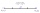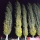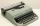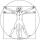# Midpoint 6

FM=8a+1, FG=42, a=? Point M is the midpoint of FG. Find unknown a.

Result

a =  2.5

#### Solution:

8*a+1 = 42/2

16a = 40

a = 52 = 2.5

Calculated by our simple equation calculator.

Leave us a comment of example and its solution (i.e. if it is still somewhat unclear...):

Showing 0 comments:Be the first to comment!#### To solve this example are needed these knowledge from mathematics:

Looking for help with calculating arithmetic mean? Looking for a statistical calculator? Do you have a linear equation or system of equations and looking for its solution? Or do you have quadratic equation? Do you want to convert length units?

## Next similar examples:

1. MG=7x-15,MG=7x-15, FG=33, x=? Point M is the midpoint of FG. Find unknown x.
2. Midpoint 5FM=3x-4, MG=5x-26, FG=? Point M is the midpoint of FG. Use the given information to find the missing measure or value.
3. Find midpointFM=5y+13, MG=5-3y, FG=? M is the midpoint of FG. Use the given information to find the missing measure or value.
4. Unknown numberIdentify unknown number which 1/5 is 40 greater than one tenth of that number.
5. PoplarHow tall is a poplar by the river, if we know that 1/5 of its total height is a trunk, 1/10th of the height is the root and 35m from the trunk to the top of the poplar?
6. SeamstressThe seamstress cut the fabric into 3 parts. The first part was the eighth fabric, the second part was three-fifths of the fabric and the third part had a length of 66 cm. Calculate the original length of the fabric.
7. Simple equationSolve for x: 3(x + 2) = x - 18
8. EquationSolve the equation: 1/2-2/8 = 1/10; Write the result as a decimal number.
9. Arithmetic averageThe arithmetic mean of the five numbers is exactly 8. The sum of these four numbers is 30. What is the fifth number?
10. 1.5 divided1.5 divided by 1 = w divided by 4
11. AssistantAssistant rewrote 15% of the entire manuscript in 12 hours. How many hours must still write to rewrite the rest of the manuscript?
12. Boys and girlsThere are 48 children in the sports club, boys are 10 more than girls. How many girls go to the club?
13. Proportionwrite the following proportion 8 is to 64 as to 2 is to x
14. CandyPeter had a sachet of candy. He wanted to share with his friends. If he gave them 30 candies, he would have 62 candies. If he gave them 40 candies, he would miss 8 candies. How many friends did Peter have?
15. Percentage - fractionsAbout what percentage we must increase number 1/6 to get number 1/3?
16. TreesA young tree is 16 inches tall. One year later, it is 20 inches tall. What is the percent increase in height?
17. AverageThe arithmetic mean of the two numbers is 71.7. One number is 5. Calculate the second number.